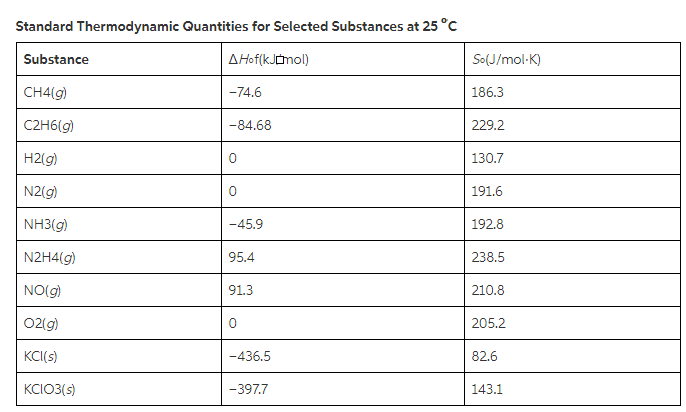# Problem: For the reaction:2KClO3(s) → 2KCl(s) + 3O2(g)Calculate ΔGºrxn at 25ºC.Express your answer using one decimal place.

###### FREE Expert Solution

We’re being asked to determine the Gibbs free energy (ΔG°rxn) at 25°C for the given reaction:

2 KClO(s) → 2 KCl (s) + 3 O(g)

We’re given the ΔH˚f and S˚ of each reactant and product:

 Substance ΔH˚f (kJ/mol) S˚ (J/mol • K) KClO3 (s) –397.7 143.1 KCl (s) –436.5 82.6 O2 (g) 0 205.2

We can use the following equation to solve for ΔG˚rxn:

$\overline{){\mathbf{\Delta G}}{{\mathbf{°}}}_{{\mathbf{rxn}}}{\mathbf{=}}{\mathbf{\Delta H}}{{\mathbf{°}}}_{{\mathbf{rxn}}}{\mathbf{-}}{\mathbf{T\Delta S}}{{\mathbf{°}}}_{{\mathbf{rxn}}}}$

For this problem, we need to do the following steps:

Step 1: Calculate ΔH˚rxn.

Step 2: Calculate ΔS˚rxn.

Step 3: Use ΔH˚rxn and ΔS˚rxn to calculate for ΔG˚rxn.

84% (70 ratings)###### Problem Details

For the reaction:

2KClO3(s) → 2KCl(s) + 3O2(g)

Calculate ΔGºrxn at 25ºC.